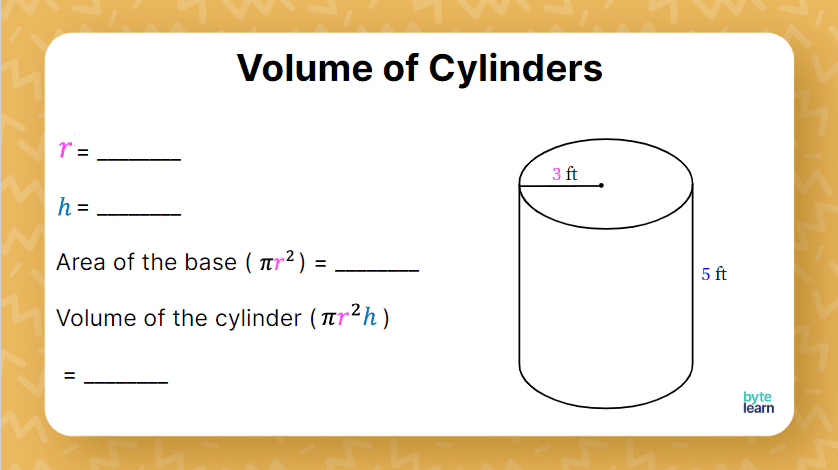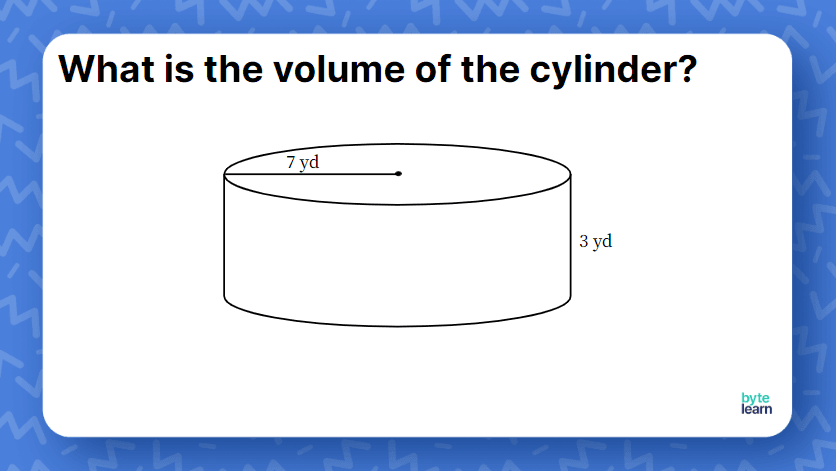ResourcesTestimonials
Plans
ResourcesTestimonials
PlansLesson plan

# Volume of a Cylinder Lesson Plan

## Overview

In this lesson, students will learn how to find the volume of a cylinder. Students will review the volume of rectangular prisms and area of circles. Then, students will make connections between rectangular prisms, circles, and cylinders to help them find the volume of a cylinder. You can expect this lesson with practice to take one 45-minute class period.

Volume
8.G.C.9

## Objective

Students will be able to find the volume of a cylinder.

## Materials

• Teacher slideshow
• Online Practice

## How to Teach Finding the Volume of a Cylinder

### Warm-up

Before having students find the volume of a cylinder, you will want to review what they already know about circles and volume. Later as we discover the formula for volume of a cylinder, the knowledge of volume of a rectangular prism and area of a circle will be helpful.When reviewing these problems, it’s important to ensure students understand that the volume of any regular prism is the area of the base times the height. If needed, use the first problem for reference. Students should also understand how to find the area of a circle, either rounded or written in terms of pi. For some students, you may need to reinforce how to write their answer in terms of pi.

### Introducing cylinders

Ask students to write down 5 things they know about a cylinder. Also, ask them to make a drawing of an object that looks like a cylinder.  Let students share what they wrote with the class and make a list on the board. This will help you capture their current understanding and build on it. It will also help to make connections to real-world objects.

### Discover the formula for volume of a cylinder

Present a cylinder to students and ask them to figure out how they can find the volume of a cylinder. Students should work in pairs to do this.

It is quite likely that a bunch of students might come up with an informal way for finding the volume of a cylinder. They might not be able to use the right words, but discovering an informal formula will help them make sense of the actual formula.

Students are likely to see that the base and height of the cylinder are like those of the rectangular prism - but instead of a rectangle as a base, you now have a circle as a base. They are likely to reason that in a rectangular prism, we multiplied the area of the base by the height - it might make sense to do the same in a cylinder!

### Vocabulary and formula

Students are now ready to understand the formula of a cylinder as it is traditionally presented. Use the same cylinder as you did earlier so that they see the connections.

Now work through an example with the class. The first problem could have scaffolding that will make it simpler for students to find the volume of the cylinder.### Class practice

Work through one more problem with the class - this time, with less scaffolding. You could ask students to leave their answers in terms of \pi for now, so it is easier to check which values were multiplied. Remind students that the units for volume are cubed.Encourage students to focus on the formula as “base area \times height” because it will help them when they have to find the volume of more unusual prisms in high school. Helping solidify that “base area” will change based on the prism can help reduce students’ confusion later, but some students may still prefer to think of the formula as V =\pi r^2h.

### Possible misconceptions

It is important to recognize that some students may not square their radius correctly, or they will multiply the height and radius before squaring. By allowing students time to work on the problem and then compare their answers with a partner or table group, you may find that students are able to correct each other with minor misconceptions.

### Finding volume of a cylinder given diameter

Now, introduce a complication - show a cylinder with the diameter given. Give students time to work through the problem.

Students can review their answers with a partner or table group. As students are working, you may notice that some students do not immediately recognize they were given the diameter first instead. Although this misconception will need to be addressed, see if students can correct each other when comparing answers.

### Example given a description

Though this example is not significantly different in terms of the information given, it might confuse some students. If students are stuck, encourage them to draw a cylinder and annotate the figure with the given measurements. If students aren’t sure how to draw one, you can let them know they just draw two ovals with a gap and parallel to each other, then connect the outer edges.

## Volume of a Cylinder Practice

Now it’s time for some independent practice! You can assign a ByteLearn online practice to your class using the link below. Students will get immediate feedback and step-by-step help if they need it. Set a due date and allow students to finish the assignment for homework. Once complete, you’ll see detailed reports of students who may need additional support, students who are ready for a challenge, and other interesting insights!Volume of a Cylinder Practice
Problem 1 of 5
<p>What is the volume of the cylinder? </p><highlight data-color="#666" data-style="italic">Enter the exact answer in terms of pi or use 3.14 for pi.</highlight><Cylinder3D data-props='{ "radius": 11, "height": 16, "unit": "cm", "heightLabel": "16 cm", "diameterLabel": "22 cm", "interactive": false, "zoom": 17, "heightLabelOffset": 0.5}'></Cylinder3D>

View this practice